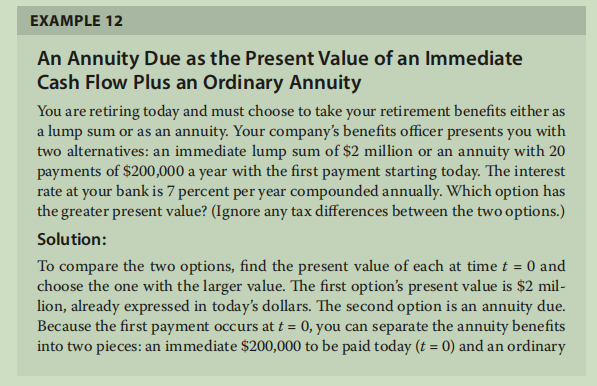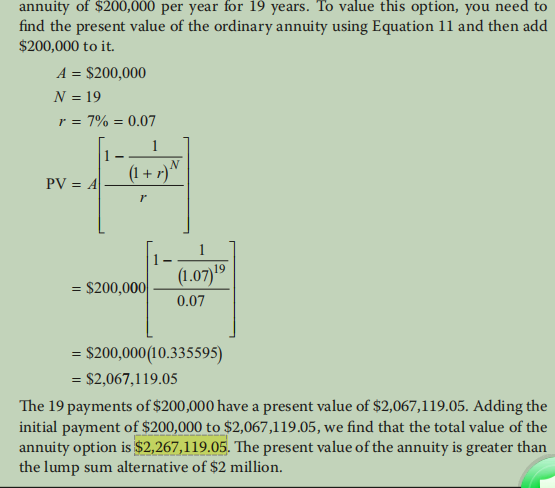# Textbook P349 simple calculation that confuses me!

Hi! I have 2 questions regarding this simple problem:If I use my Texax Instruments BAII Plus calculator to enter the following: (under ordinary annuity mode)

N:19
I/Y: 7
PMT: 200,000
FV: 200,000 (actually the result is the same without entering the FV)

When computing PV, I get the result as 2,122,420.715. The textbook calculation for this step is 2,067,119.05. I understand the formula used in the textbook but I wonder why using the calculator yields a different result?

My second question is：a different approach will be viewing this as an annuity due (payment starting today of \$200000 for the next 20 years), so under the mode of “BGN” of the calculator, if we enter the following:

N:20
I/Y: 7
PMT: 200,000
FV: 200,000

Then when computing PV, I get the result of 2,318,802.849. But the textbook’s result is 2,267,119.05. I’m totally lost!

Anyone can arrive at the same result as me? Any answer will be greatly appreciated!

I solved the calculation by replacing FV with zero for both “Ordinary” and “Due” mode. But another question is：if we go by the approach in the textbook, assume this is an ordinary annuity with 19 payments (only to add the first \$200,000 at the end),shouldn’t the FV be \$200,000 instead of zero, as the 19th payment is \$200,000? Any thoughts? Thank you!

Problem solved again!

Explanation to the above question: as \$200,000 is already counted when “PMT” is entered as 19, so it shouldn’t be counted again.

2 Likes